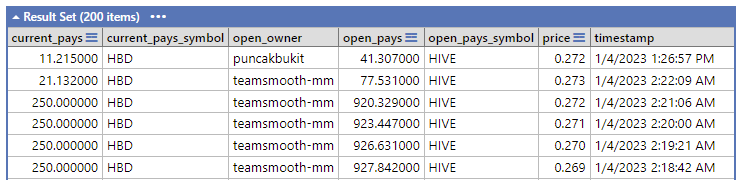# 每天进步一点点：MS-SQL保留指定位数小数

in HIVE CN 中文社区9 days ago(图源 ：pixabay)

``````a = 1.1118
b = round(a, 3)
print(b)
``````

``````a = 1.1118
print(f'{a:.3f}')
``````

`select round(0.3694826767916468913, 3);`

Reveal spoiler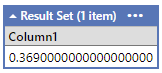Reveal spoiler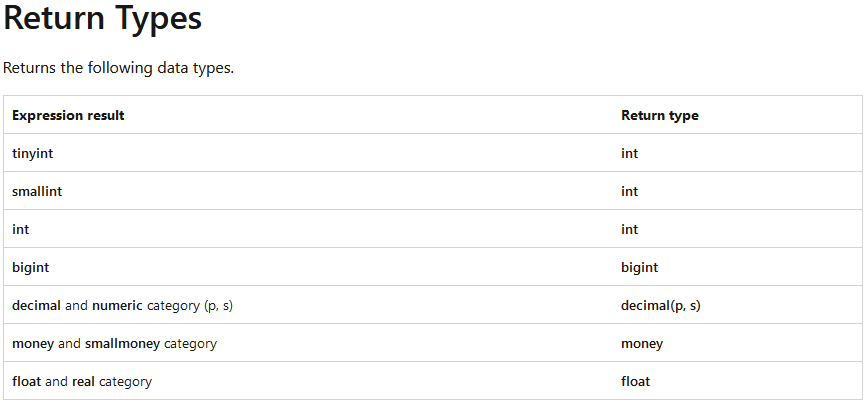`SELECT TRUNCATE(135.376, 2);`

`CAST ( expression AS data_type [ ( length ) ] )`

`SELECT CAST (0.3694826767916468913 AS decimal (18,3));`

Reveal spoiler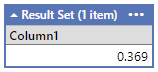``````select top 200 current_pays, current_pays_symbol, open_owner, open_pays, open_pays_symbol,
CAST(current_pays/open_pays AS decimal (18,3))as price, timestamp
from VOFillOrders
where current_owner='oflyhigh'
order by timestamp desc
``````

`amount_to_sell/min_to_receive as price`

`CAST(current_pays/open_pays AS decimal (18,3))as price`

Reveal spoiler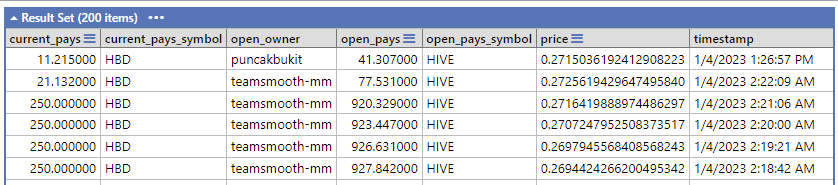Reveal spoiler